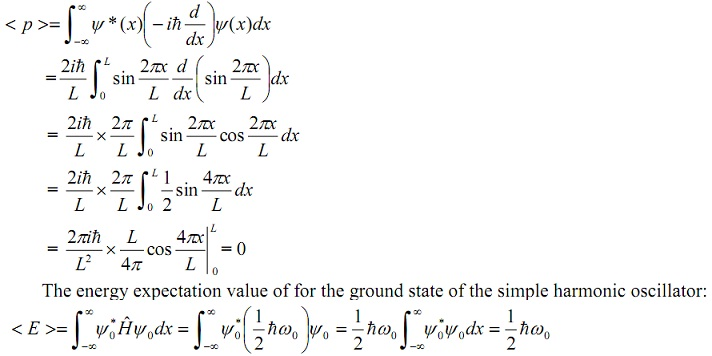#### Operators, Physics tutorial

Introduction to Operators:

The operators are quite significant in Quantum mechanics as each and every observable is represented through a Hermitian operator. The Eigen-values of the operator are the possible values the physical observable can take, and the expectation value of the observable in any specific state is the average value it takes in that specific state. Commuting operators point out that the corresponding physical observables can have the similar Eigen-states, or equivalently, they can both be measured concurrently by infinite accuracy.

Linear Operators:

A linear map or linear transformation or linear operator, is a function f: X → Y between the vector spaces X and Y which preserves vector addition and scalar multiplication, that is,

f (x1 + x2) = f(x1) + f(x2)

f (λx) = λ f(x) for λ ∈ K, a constant, and x1, x2 ∈ X

Equally, f (ax1 + bx2) = af (x1) + bf(x2)

As an illustration, the differential operator is a linear operator.

d/dx (α f1(x) + β f2(x)) = α d/dx f1(x) + β d/dx f2 (x)

Here α and β are constants (that is, scalars) in the underlying field.

Eigen-values of a Linear Operator:

Assume that 'A' be an operator and 'c' the associated Eigen-value corresponding to the Eigenvector 'ψ'. Then, we can represent:

Aψ = λψ

Often, the operator 'A' is a matrix, and the Eigen-vector ψ a column matrix. It obeys that:

(A - λI) ψ = 0

Here 'I' is the suitable identity matrix, that is, a square matrix that consists of 1 all along its main diagonal and zero elsewhere.

For a non-trivial solution, we need that the determinant disappear, that is,

|A - λI| = 0

Resolving the resultant feature (or secular) equation, we get the possible values of λ, termed as the Eigen-values. Then armed by the Eigen-values, we can then get the associated Eigen-functions.

Expectation value:

The expectation value of a quantity is the statistical expected mean value of all the measurements.

The (statistical) average value of the numbers x1, x2... xn is x‾ = 1/n i=1Σn xi. Though, if there is a distribution, such that there are fi of the value xi, i = 1, 2, 3....n then the average becomes

x‾= [i=1Σm fixi]/[ i=1Σm fi] = 1/n i=1Σm fixi, as i=1Σm fi = n

As 'n' is the total number of observations.

In case of quantum mechanics, the average value or the expectation value of the operator is:

< . > = -∞∫∞ ψ* (x) (.) ψ (x) dx

Therefore, the expectation value of x is as:

< x > = -∞ ψ* (x) x ψ (x) dx

Therefore, if ψ = √ (2/L) sin (2πx/L), having n = 2, and 0 < x < L,

< x > = 2/L 0L sin (2πx/L) x sin (2πx/L) dx

< x > = 2/L 0L x sin2 (2πx/L) dx

< x > = 2/L x L2/4 = L/2

The expectation value of the momentum for the similar case above is as follows:As ψo is normalized, this is a special case of the common result

< α > = -∞ ψ* α ‾ ψ dx = λ -∞ ψ* ψ dx = λ

Therefore, we observe that for any Eigen-state of an operator, the expectation value of the observable symbolized by that operator is the Eigen-value.

More commonly, we would write the expectation value of the operator, 'A', in a certain state 'ψ', as

<ψ |A| ψ>

Commutators and simultaneous Eigen-states:

Let suppose an operator Pˆ that symbolizes a physical observable of a system, example: energy or momentum. Assume that the state 'ψ' consists of a particular value 'p' of this observable, that is, Pˆ ψ = P ψ. Assume further that the similar state as well consists of the value 'q' of  a second observable represented by the operator Qˆ, that is, Qˆ ψ = q ψ. Then p and q are known as simultaneous Eigen-values. Then,

Qˆ Pˆ ψ = Qˆ p ψ = p Qˆ ψ = p q ψ

Likewise,

Pˆ Qˆ ψ = Pˆ q ψ = q Pˆ ψ = q p ψ

As 'p' and 'q' are just real numbers, then qp = pq. Therefore, the condition for simultaneous Eigen-states is that Pˆ Qˆ ψ = Qˆ Pˆ ψ or

Pˆ Qˆ = Qˆ Pˆ = 0

Pˆ Qˆ = Qˆ Pˆ is stated to be the commutator of Pˆ and Qˆ and operators that satisfy the condition Pˆ Qˆ = Qˆ Pˆ = 0 are stated to commute. The commutator is generally written as [Pˆ Qˆ]

Matrix Elements of a Linear Operator:

We can symbolize any operator 'A' through a square n x n matrix:

Aij = < Φi |A| Φj >, i, j = 1, n

Change of Basis:

Assume that 'V' be a subspace of Rn for some 'n'. A collection B = {v1, v2, ..., vr } of vectors from 'V' is stated to be a basis for 'V' when B is linearly independent and spans 'V'. If either one of such criteria is not satisfied, then the collection is not a basis for 'V'. If a collection of vectors spans 'V', then it includes enough vectors so that each and every vector in 'V' can be written as the linear combination of such in the collection. Whenever the collection is linearly independent, then it does not have so many vectors that a few become dependent on the others. Intuitively, then, a basis has merely the right size: It is big adequate to span the space however not so big as to be dependent.

Tutorsglobe: A way to secure high grade in your curriculum (Online Tutoring)